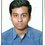# Solving A Kind Of Diophantine Equation

I have seen many questions like this $\large{\dfrac{1}{x} + \dfrac{1}{y} = \dfrac{1}{n}}$ where n is any positive integer, Find all the ordered pair (x,y).

So How to solve these kinds of questions?

Lets Start.

See,

$\large{\dfrac{1}{x} + \dfrac{1}{y} = \dfrac{1}{n}}$

$\implies \large{\dfrac{x+y}{xy} = \dfrac{1}{n}}$

$\implies \large{ x+y = \dfrac{xy}{n}}$

$\implies \large{ nx+ny = xy}$

$\implies \large{ nx+ny-xy = 0}$

$\implies \large{ x(n-y)+ny = 0}$

$\implies \large{ x(n-y)+ny -n^2= -n^2} \space \space \space \text{Adding }-n^2 \text{ to both sides}$

Now Factorizing it , will give

$\implies \large{(x-n)(y-n)=n^2}$.

Say $\large{n=p_{1}^{a_{1}} p_{2}^{a_{2}}...}$ where $p_{1},p_{2}$ are primes.

So total number of divisors of $n = (a_1 +1)(a_2 +1)..(a_n+1)$

So Number of pairs = No. of divisors = $(a_1 +1)(a_2 +1)..(a_n+1)$

PRACTICE EXAMPLE

• $\large{\dfrac{1}{x} + \dfrac{1}{y} = \dfrac{1}{2015}}$ , Find all the ordered pair (x,y).

• $\large{\dfrac{1}{x} + \dfrac{1}{y} = \dfrac{1}{1890}}$ , Find all the ordered pair (x,y).

Lets END Here.

Hope it helpsNote by Md Zuhair
3 years, 10 months ago

This discussion board is a place to discuss our Daily Challenges and the math and science related to those challenges. Explanations are more than just a solution — they should explain the steps and thinking strategies that you used to obtain the solution. Comments should further the discussion of math and science.

When posting on Brilliant:

• Use the emojis to react to an explanation, whether you're congratulating a job well done , or just really confused .
• Ask specific questions about the challenge or the steps in somebody's explanation. Well-posed questions can add a lot to the discussion, but posting "I don't understand!" doesn't help anyone.
• Try to contribute something new to the discussion, whether it is an extension, generalization or other idea related to the challenge.

MarkdownAppears as
*italics* or _italics_ italics
**bold** or __bold__ bold
- bulleted- list
• bulleted
• list
1. numbered2. list
1. numbered
2. list
Note: you must add a full line of space before and after lists for them to show up correctly
paragraph 1paragraph 2

paragraph 1

paragraph 2

[example link](https://brilliant.org)example link
> This is a quote
This is a quote
    # I indented these lines
# 4 spaces, and now they show
# up as a code block.

print "hello world"
# I indented these lines
# 4 spaces, and now they show
# up as a code block.

print "hello world"
MathAppears as
Remember to wrap math in $$ ... $$ or $ ... $ to ensure proper formatting.
2 \times 3 $2 \times 3$
2^{34} $2^{34}$
a_{i-1} $a_{i-1}$
\frac{2}{3} $\frac{2}{3}$
\sqrt{2} $\sqrt{2}$
\sum_{i=1}^3 $\sum_{i=1}^3$
\sin \theta $\sin \theta$
\boxed{123} $\boxed{123}$

Sort by:

Hey, can you elaborate a bit more? Like for the practice example 1, no of divisors is 3..Then how do you proceed ?

- 3 years, 10 months ago

Like the way I proceeded.

- 3 years, 10 months ago

So let me help u with the 1st one. Try the 2nd on ur own

- 3 years, 10 months ago

$\large{\dfrac{1}{x} + \dfrac{1}{y} = \dfrac{1}{2015}}$

$\implies \large{\dfrac{x+y}{xy} = \dfrac{1}{2015}}$

$\implies \large{ x+y = \dfrac{xy}{2015}}$

$\implies \large{ 2015x+2015y = xy}$

$\implies \large{ 2015x+2015y-xy = 0}$

$\implies \large{ x(2015-y)+2015y = 0}$

$\implies \large{ x(2015-y)+2015y -2015^2= -2015^2} \space \space \space \text{Adding } -2015^2 \text{ to both sides}$

Now Factorizing it , will give

$\implies \large{(x-2015)(y-2015)=2015^2}$.

Now see that if the in the place of $2015^2$ it was $2 \times 3$ (SAY).

Then $\implies \large{(x-2015)(y-2015)=2*3}$.

So the solution would have been $x-2015=2 \space \space \space y-2015=3$ or $x-2015=3 \space \space \space y-2015=2$ or $x-2015=6 \space \space \space y-2015=1$ or $x-2015=1 \space \space \space y-2015=6$.

So here we can see that number of divisors of 6 are 4(namely 1,2,3,6).

So here we have 4 solns.

But what in case of 2015^2?

We will get $2015^2=p_{1}^{a_1} p_{2}^{a_2}...$ So

No. of divisors = No of Solutions = $(a_1+1)(a_2+1)...$

OK?

- 3 years, 10 months ago

Ok thanks..

- 3 years, 10 months ago

@Md Zuhair- You also have to account for the negative divisors ,thus the total number of solutions will be $2(\text{number of divisors})-1$,Assuming x and y are integers thus incorporating negative values for x and y

where $(0,0)$ is the neglected solution

- 3 years, 9 months ago

@Md Zuhair I think that the number of ordered pairs are the number of factors of $n^2$

So the no. of ordered pairs would be $(2a_1+1)(2a_2+2)..(2a_n+1)$

- 3 years, 4 months ago